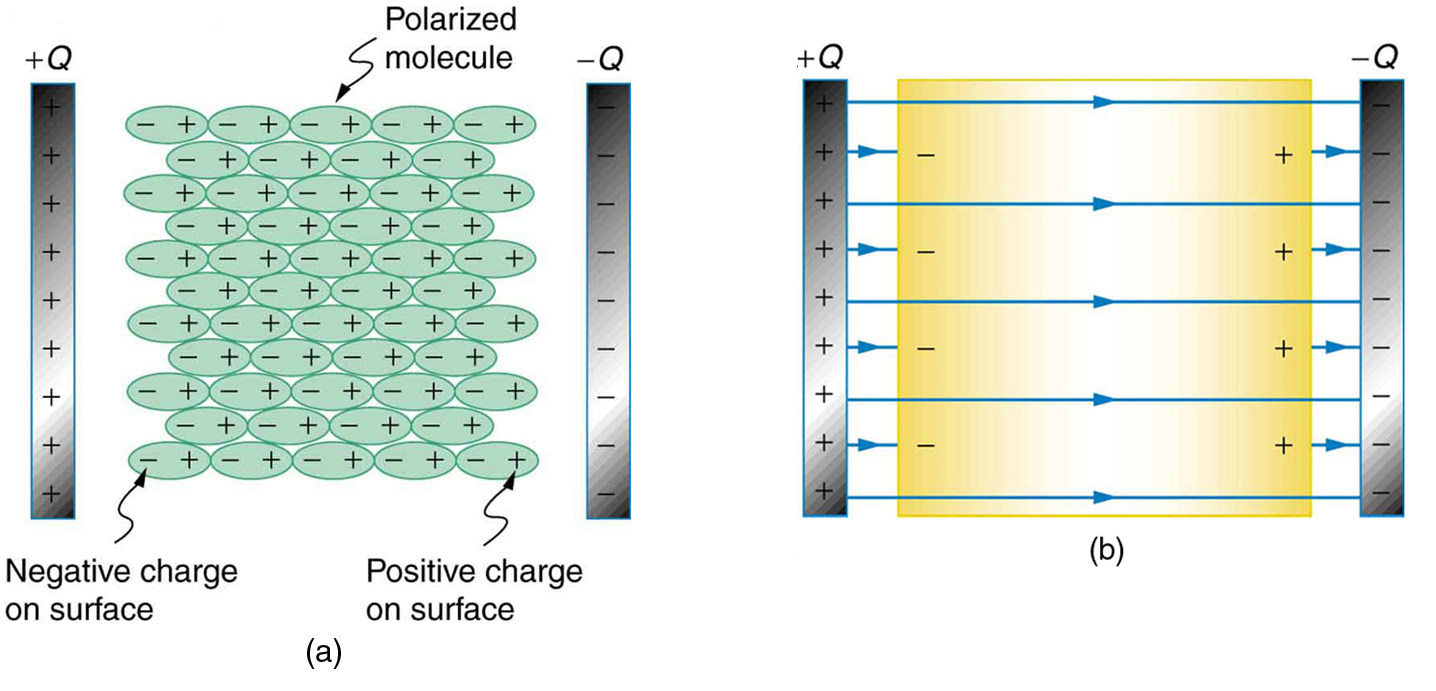# Capacitor and Its Principle

• The charge can stay in the body for some time when the charge is given to that body.
• The time for which a body can store charge depends on the shape of the body.
• If the body has pointed ends or sharp edges, the charges leak through them. If the body is round, the charges can stay for longer time.
• It is also found that different bodies can store different amount of charges.
• In other words, the different bodies have different charge holding capacity, which is called the capacitance (or capacity).
• All bodies whatever we deal with have charge storing capacity to some extent.
• Those bodies that have been designed especially to store charges are called capacitors.
• Basically, a capacitor has two conducting surfaces separated by an insulating medium called dielectric. The conducting surfaces are called plates of the capacitor.
• The circuit symbol of capacitor is represented by two parallel vertical lines that indicate two conducting plates and a horizontal line attached to each plate which is the lead for connecting it to the external circuit.

Principle of a Capacitor

Let us consider a dielectric medium placed between two metallic plates A and B forms a capacitor. The plate A is connected to the anode and the plate B is connected to the cathode.

• The dielectric medium placed between the two plates can be considered to be consisting of a large number of thin layers. Only a few layers have been shown in the figure.• Since the plate A is connected to the anode of the battery, it is charged positively by conduction.
• Due to the positive charge on the plate A, negative charge is induced on the left side and the positive charge is induced on the right side of the first layer of dielectric.
• Due to the positive charge on the right side of the first layer of dielectric, negative charge is induced on the left side and positive charge is induced on the right side of the second layer of the dielectric and so on.
• As a result, the left side of the metal plate B will have induced negative charge and the right side will have induced positive charge.
• The induced positive charges on the right side of the plate B are the free charges. Hence, they are neutralized by the electron coming from the cathode of the battery.
• However, the negative induced charges on the left side of the plate B are bound charges. Hence, the plate A of the capacitor is charged positively and the plate B of the capacitor is charged negatively.
• The charges in the plates of the capacitor stay in them even if the battery is disconnected. Hence, a capacitor can store charges. This is the principle of a capacitor.
• It is important to note that if +q is the positive charge stored in the plate A of the capacitor, the charge stored in the plate B of the capacitor is –q.
• In other words, the two plates of a capacitor will have the same amount of opposite charges.

Capacitance of a Capacitor

• The capacitance of a capacitor is its ability to store charges.
• If a charge is given to a capacitor, the potential difference across its plate rises.
• Conversely, if the potential difference across the plates of a capacitor rises, the charge stored in it also increases.
• If ‘q’ is the charge sored in a capacitor when its potential difference is ‘V’,

Then, we have q ∝  V

q= CV

Where ‘C’ is the proportionality constant called the capacitance of the capacitor. The capacitance (C) of the capacitor does not depend upon the material of the plates. However, the capacitance of the capacitor is the function of the nature of the dielectric medium.

Therefore, we have C = q/V

If V = 1 unit, C = q

Hence, the capacitance of the capacitor can also be defined as the charge to be given to the capacitor in order to raise its potential difference by unity.

Unit of Capacitance

We have C= q/v. In SI system, ‘q’ is in Coulomb and ‘v’ is in volt.

Hence, the SI unit of capacitance is Coulomb/volt. That is C = Coulomb/volt = c/V = cV-1

The unit of capacitance is called farad (F). That is 1 farad = 1 cV-1

A capacitor is said to have one farad capacitance if its potential difference rises by one volt when a charge of one coulomb is given to it.

i.e., C = 1 farad if q = 1 c and V = 1 volt.

Factors Determining the Capacitance of a Capacitor

• The following are the factors on which the capacitance of a capacitor depend on:
1. The area of the metallic plates of the capacitor.
2. The distance between the metallic plates.
3. The permittivity of the insulating medium (or dielectric).

References: